### Home > AC > Chapter 14 > Lesson 14.3.1.4 > Problem3-60

3-60.
1. Factor each expression below. Homework Help ✎

1. x2 + 8x

2. 6x2 + 48x

3. 2x2 +14x −16

4. 2x3 −128x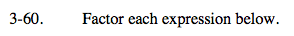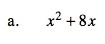x(x + 8)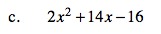Factor out the 2 first.

2(x + 8)(x − 1)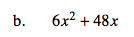6x is a common factor.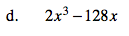2x is a common factor. Factor it out and then factor again.